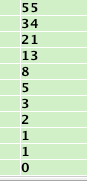# Project 5x: Assembly Code Challenges (30 points)

## Challenge 1: Secret Message (10 pts)

The code loads encrypted values onto the stack, decrypts them to place a secret message in ASCII into memory, and then erases the memory.

Use Jasmin to run the code until it has decrypted the message, but has not erased it yet, and capture a whole-desktop image of the decrypted RAM contents. Save the image as "Proj 5xa from YOUR NAME".

Convert the message to ASCII and type it in the body of your email message.

## Challenge 2: Fibonacci Numbers (20 pts.)

Write an assembly language program to display the first 10 Fibonacci numbers, as shown below:Each Fibbonacci number is the sum of the first two previous ones, and the first two are:

F0 = 0
F1 = 1

F2 = F1 + F0 = 1
F3 = F2 + F1 = 1 + 1 = 2
F4 = F3 + F2 = 2 + 1 = 3
...

Tip: You can learn how to use loops here:

Turn in a screen shot showing your results, and attach an ASM file of your code, saved from Jasmin.